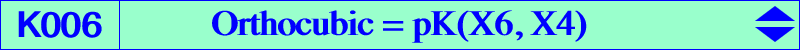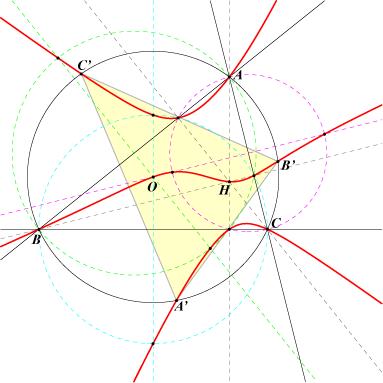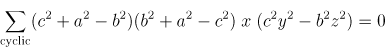X(1), X(3), X(4), X(46), X(90), X(155), X(254), X(371), X(372), X(485), X(486), X(487), X(488), X(6212), X(6213), X(8946), X(8947), X(8948), X(8949) vertices of orthic triangle excenters A', B', C' on the circumcircle and the midpoints A1, B1, C1 of A'B'C'. X3-OAP points, see Table 53 and their isogonal conjugates six intersections of a perpendicular bisector and the circle with diameter the corresponding side of ABC i.e. the centers of the six squares erected externally or internally on the sides of ABC. See Table 32 : Kiepert triangles and related curves.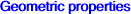The Orthocubic is the isogonal pK with pivot H = X(4). See Table 27. It is also the isogonal pK with pivot O with respect to the orthic triangle. It is a member of the class CL043 : it meets the circumcircle at A, B, C and three other points A', B', C' where the tangents are concurrent at the point X(25). See further details below and also Q063. The orthocubic of A'B'C' is K376. K006 is also related to the stelloid Q083. Its isotomic transform is pK(X76, X264). The symbolic substitution SS{a → √a} transforms K006 into K1082. Locus properties : Let P be a point and PaPbPc its pedal triangle. A1 is the reflection of P in the line PbPc and B1, C1 are defined similarly. The triangles ABC and A1B1C1 are perspective if and only if P lies on the orthocubic (together with the line at infinity and the circumcircle). Let A1B1C1 be the prepedal/antipedal triangle of P and A2, B2, C2 the reflections of P through the sides of ABC. The locus of P such that A1B1C1 and A2B2C2 are perspective is the orthocubic (together with the line at infinity and the circumcircle). (Floor van Lamoen) Locus of point P such that P, GSC(P), X(155) are collinear. (X(155) is the orthocenter of the tangential triangle). GSC is defined here. Locus of point P such that the reflection triangles of P and P* (isogonal conjugate of P) in the sidelines of ABC are perspective. The perpendicular at P to BC meets the line AP* at A1. La is the parallel at A1 to AP. Define B1, C1 Lb, Lc similarly. These lines La, Lb, Lc concur if and only if P lies on the union of the McCay cubic and the orthocubic. Now, if La is the parallel at A1 to BC and if Lb, Lc are defined likewise, the lines concur if and only if P lies on the quartic Q023 (Floor van Lamoen and friends, Hyacinthos #5360 & sq.). Locus of M whose orthotransversal and polar in the circumcircle are parallel. With perpendicular, we find the quartic Q110.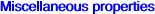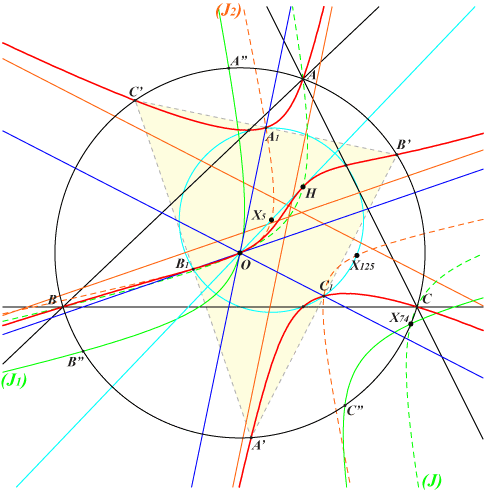Asymptotes and related topics The asymptotes of K006 are parallel to the Simson lines passing through O. These Simson lines are those of the three points A", B", C" on (O) and on the hyperbola (J1) which is the image of the Jerabek hyperbola (J) under the translation mapping H onto O. The reflections A', B', C' of A", B", C" about O are the intersections of (O) and K006. More details below. The Simson lines meet K006 again at three points A1, B1, C1 which are the midpoints of A'B'C' and also the midpoints of HA", HB", HC" and therefore lying on the nine point circle and on the rectangular hyperbola (J2), the homothetic of (J1) under h(H, 1/2). (J1) contains : X(3), X(20), X(74), X(2574), X(2575) (J2) contains : X(3), X(5), X(125), X(182), X(2574), X(2575)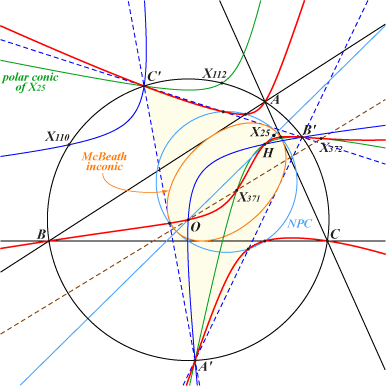Intersection with the circumcircle Recall that the points A', B', C' are the intersections (apart A, B, C) of K006 and the circumcircle. These points lie on several remarkable rectangular hyperbolas : • (H1) passing through X(3), X(4), X(110), X(155), X(1351), X(1352), X(2574), X(2575), • (H2) passing through X(4), X(76), X(99), X(376), X(487), X(488), • the polar conic of X(25) passing through X(4), X(112), X(371), X(372), X(378), X(1064). These form a pencil and each hyperbola meets K006 at H, A', B', C' and two other points which are H-Ceva conjugates. *** ABC and A'B'C' both circumscribe the MacBeath inconic with foci O and H. These triangles share the same Euler line and the same nine point circle.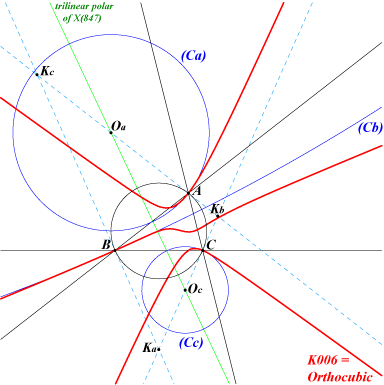Osculating circles The centers of the three osculating circles at A, B, C to this cubic are collinear on the trilinear polar of X(847). More generally, the locus of pivots of all isogonal pKs with the same property is the nonic Q031.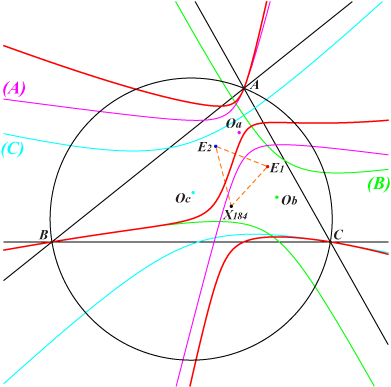Polar conics of A, B, C Let (A), (B), (C) be the polar conics of A, B, C in K006. Denote by Oa, Ob, Oc their respective centers. Oa = b^2c^2 / SA : b^4 / SC : c^4 / SB. The isogonal conjugate Oa* of Oa is the intersection of the line AX(264) and the parallel at O to BC. Oa* = a^2 SA : c^2 SC : b^2 SB The triangles ABC and OaObOc are triply perspective at X(184), E1 and E2. These two latter points are the orthocorrespondents of the Brocard points Ω1 and Ω2. Note that OaObOc is also triply perspective with the cevian, anticevian, circumcevian triangles of the Lemoine point K.Furthermore, OaObOc is perspective • to the cevian triangle of any point on pK(X184 x X1987, X1987) passing through X(3), X(6), X(184), X(217), X(237), X(436), X(1298), X(1987) and X(4) x X(401), • to the anticevian triangle of any point on pK(X25, X4 x X401) passing through X(4), X(6), X(98), X(184), X(232), X(1987), X(2052), X(3164) and X(4) x X(401). In both cases, the locus of the perspector is pK(X25, X436) passing through X(2), X(25), X(51), X(184), X(275), X(436), X(1988), X(2052), X(3168). The two former cubics generate a pencil that contains a third pK namely pK(X6 x X1971, X184) passing through X(6), X(54), X(184), X(1971), X(1976), X(1987) and X(4) x X(401). The three cubics contain A, B, C, X(6), X(184), X(1987), X(4) x X(401) and two imaginary points on the circumcircle and on the Lemoine axis. These points are the isogonal conjugates of the infinite points of the Steiner ellipses. These properties are generalized in CL059. Note that the four cubics mentioned above are all anharmonically equivalent.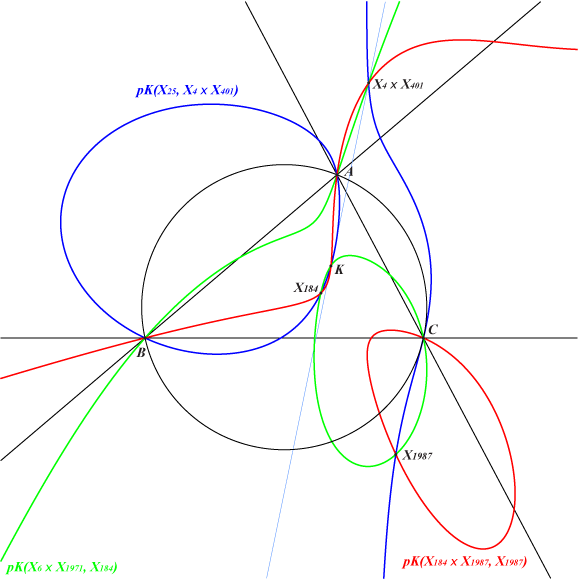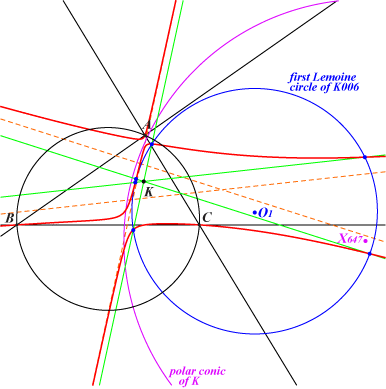Lemoine circles in the Orthocubic There is one and only one point whose polar conic in K006 is a circle and this point is the Lemoine point K. K006 is the only pivotal isogonal cubic having this property. See K1237 for a generalization and other cubics. This polar conic is the circle with center X(647) passing through X(15), X(16). It is orthogonal to the circumcircle and belongs to the pencil of coaxial circles containing the Parry circle and the three Apollonius circles.. The parallels to the asymptotes of K006 passing through K meet K006 again at six points that lie on a circle (C) whose center O1 is the midpoint of K, X(647). This circle is orthogonal to the circle with center O and radius R√(1-tan2Ω) where Ω is the Brocard angle. This circle (C) is analogous to the first Lemoine circle obtained when K006 is replaced by the cubic which is the union of the sidelines of ABC. *** The asymptotes of K006 are now reflected about K giving three other lines meeting K006 again at six other points lying on a same circle with center O2. This circle is analogous to the second Lemoine circle obtained when K006 is replaced by the cubic which is the union of the sidelines of ABC. *** Tucker circles in the Orthocubic The properties above can be generalized as follows. The homothety with center X(6), ratio k, transforms the asymptotes of K006 into three lines meeting K006 again at six points lying on a same circle which is analogous to a Tucker circle. The values k = 0, k = -1 give the two Lemoine circles above.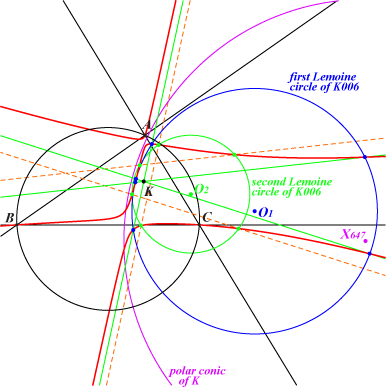When k = 1, we obtain the satellite of the line at infinity since the circle must decompose. All these circles have their centers on the line X(6), X(647) which is in a way the Brocard axis of the cubic K006.pKs anharmonically equivalent to K006 pK(Ω = p : q : r, P = u : v : w) and K006 are equivalent if and only if ∑ a^2 (b^2 - c^2) SA^2 u^2 / p = 0 This may be construed so that the barycentric quotient P^2 ÷ Ω lies on the trilinear polar (L) of X(6528), the isotomic conjugate of X(520). (L) passes through X(2), X(216), X(232), X(264), X(324), X(393), X(2052), X(2404), X(3164), etc. It follows that K006 is equivalent to : • pK(P, P) if and only if P lies on the line (L) above. • pK(X6, P) if and only if P lies on the diagonal rectangular hyperbola passing through X(1), X(4), X(19), X(920), X(1075), X(1249), X(1712), X(1713), X(1714), X(1715), X(2588), X(2589), X(3068), X(3069), X(3183), X(3186), etc. • pK(X2, P) if and only if P lies on the diagonal conic passing through X(2), X(4), X(92), X(1895), X(2592), X(2593), etc. Note that the complement of any such pK is also a pK, with pivot X2, equivalent to K006. • pK(Ω, X4) if and only if Ω lies on the circum-conic passing through X(2), X(6), X(25), X(37), X(42), X(111), X(251), X(263), X(308), X(393), X(493), X(494), X(588), X(589), X(694), X(941), X(967), X(1169), X(1171), X(1218), X(1239), X(1241), X(1383), X(1400), X(1427), X(1880), X(1976), X(1989), X(2054), X(2165), X(2248), X(2350), X(2395), X(2433), X(2963), X(2981), X(2987), X(2998), X(3108), X(3228), etc. • pK(Ω, X2) if and only if Ω lies on the circum-hyperbola passing through X(2), X(3), X(97), X(276), X(394), X(1073), X(1214), X(1217), X(1297), X(2416), etc. Note that the anticomplement of any such pK is also a pK, with pole X2, equivalent to K006. More generally, • for a given pole Ω, the pivot P must lie on on the diagonal conic Γ1(Ω) with equation ∑ a^2(b^2-c^2)SA^2 q r x^2 = 0. • for a given pivot P, the pole Ω must lie on on the circum-conic Γ2(P) with equation ∑ a^2(b^2-c^2)SA^2 u^2 y z = 0. Now, if Γ0 denotes the circum-hyperbola above with perspector X(520), passing through G and O, Γ2(P) is clearly the barycentric product P^2 × Γ0. Hence, for any point X ≠ G on Γ0, the barycentric product P × pK(X, G) = pK(P^2 × X, P) is a pK with pivot P equivalent to K006. The following cubics are all pKs equivalent to the Orthocubic K006 : K070a, K070b, K168, K170, K171, K233, K424a, K424b, K678, K707, K857, K1039, K1045, K1046, K1047.